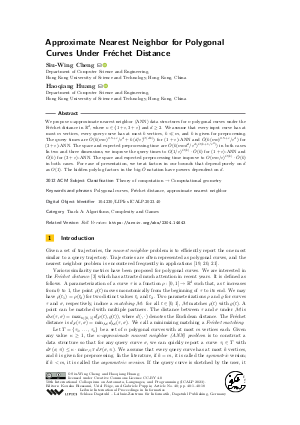Document# Approximate Nearest Neighbor for Polygonal Curves Under Fréchet Distance

### Authors Siu-Wing Cheng, Haoqiang Huang## File

LIPIcs.ICALP.2023.40.pdf
• Filesize: 0.91 MB
• 18 pages

## Cite As

Siu-Wing Cheng and Haoqiang Huang. Approximate Nearest Neighbor for Polygonal Curves Under Fréchet Distance. In 50th International Colloquium on Automata, Languages, and Programming (ICALP 2023). Leibniz International Proceedings in Informatics (LIPIcs), Volume 261, pp. 40:1-40:18, Schloss Dagstuhl - Leibniz-Zentrum für Informatik (2023)
https://doi.org/10.4230/LIPIcs.ICALP.2023.40

## Abstract

We propose κ-approximate nearest neighbor (ANN) data structures for n polygonal curves under the Fréchet distance in ℝ^d, where κ ∈ {1+ε,3+ε} and d ≥ 2. We assume that every input curve has at most m vertices, every query curve has at most k vertices, k ≪ m, and k is given for preprocessing. The query times are Õ(k(mn)^{0.5+ε}/ε^d+ k(d/ε)^O(dk)) for (1+ε)-ANN and Õ(k(mn)^{0.5+ε}/ε^d) for (3+ε)-ANN. The space and expected preprocessing time are Õ(k(mnd^d/ε^d)^O(k+1/ε²)) in both cases. In two and three dimensions, we improve the query times to O(1/ε)^O(k) ⋅ Õ(k) for (1+ε)-ANN and Õ(k) for (3+ε)-ANN. The space and expected preprocessing time improve to O(mn/ε)^O(k) ⋅ Õ(k) in both cases. For ease of presentation, we treat factors in our bounds that depend purely on d as O(1). The hidden polylog factors in the big-Õ notation have powers dependent on d.

## Subject Classification

##### ACM Subject Classification
• Theory of computation → Computational geometry
##### Keywords
• Polygonal curves
• Fréchet distance
• approximate nearest neighbor

## Metrics

• Access Statistics
• Total Accesses (updated on a weekly basis)
0

## References

1. P.K. Agarwal and J. Matoušek. Ray shooting and parametric search. SIAM Journal on Computing, 22(4):794-806, 1993.2. P.K. Agarwal, N. Rubin, and M. Sharir. Approximate nearest neighbor search amid higher-dimensional flats. In Proceedings of the European Symposium on Algorithms, pages 4:1-4:13, 2017.3. H. Alt and M. Godau. Computing the Fréchet distance between two polygonal curves. International Journal of Computational Geometry and Applications, 5:75-91, 1995.4. A. Andoni, P. Indyk, R. Krauthgamer, and H.L. Nguyen. Approximate line nearest neighbor in high dimensions. In Proceedings of the ACM-SIAM Symposium on Discrete Algorithms, pages 293-301, 2009.5. K. Bringmann, A. Driemel, A. Nusser, and I. Psarros. Tight bounds for approximate near neighbor searching for time series under Fréchet distance. In Proceedings of the ACM-SIAM Symposium on Discrete Algorithms, pages 517-550, 2022.6. S.-W. Cheng and H. Huang. Curve simplification and clustering under Fréchet distance. In Proceedings of the ACM-SIAM Symposium on Discrete Algorithms, pages 1414-1432, 2023.7. T.H. Cormen, C.E. Leiserson, R.L. Rivest, and C. Stein. Introduction to Algorithms. MIT Press, second edition, 2001.8. M. de Berg, D. Halperin, M. Overmars, J. Snoeyink, and M. van Kreveld. Efficient ray shooting and hidden surface removal. Algorithmica, 12:30-53, 1994.9. A. Driemel and I. Psarros. (2+ε)-ANN for time series under the Fréchet distance. arXiv preprint v5, 2021. URL: https://arxiv.org/abs/2008.09406.
10. A. Driemel and F. Silvestri. Locality-sensitive hashing of curves. In Proceedings of the International Symposium on Computational Geometry, pages 37:1-37:16, 2017.11. T. Eiter and H. Mannila. Computing discrete Fréchet distance. Technical Report CD-TR 94/64, Christian Doppler Laboratory for Expert Systems, TU Vienna, Austria, 1994.12. I.Z. Emiris and I. Psarros. Products of Euclidean metrics, applied to proximity problems among curves: Unified treatment of discrete fréchet and dynamic time warping distances. ACM Transactions on Spatial Algorithms and Systems, 6(4):1-20, 2020.13. A. Filtser, O. Filtser, and M.J. Katz. Approximate nearest neighbor for curves: simple, efficient, and deterministic. In Proceedings of the International Colloquium on Automata, Languages, and Programming, pages 48:1-48:19, 2020.14. S. Har-Peled. A replacement for Voronoi diagrams of near linear size. In Proceedings of the Annual IEEE Symposium on Foundations of Computer Science, pages 94-103, 2001.15. S. Har-Peled, P. Indyk, and R. Motwani. Approximate nearest neighbor: towards removing the curse of dimensionality. Theory of Computing, 8:321-350, 2012.16. P. Indyk. Approximate nearest neighbor algorithms for Fréchet distance via product metrics. In Proceedings of the Annual Symposium on Computational Geometry, pages 102-106, 2002.17. P. Indyk and R. Motwani. Approximate nearest neighbor: towards removing the curse of dimensionality. In Proceedings of the Annual ACM Symposium on Theory of Computing, pages 604-613, 1998.18. Mirzanezhad M. On the approximate nearest neighbor queries among curves under the Fréchet distance. arXiv preprint, 2020. URL: https://arxiv.org/abs/2004.08444.
19. C. Shahabi, M. Kolahdouzan, and M. Sharifzadeh. A road network embedding technique for k-nearest neighbor search in moving object databases. GeoInformatica, 7:255-273, 2003.20. Z. Song and N. Roussopoulos. K-nearest neighbor search for moving query point. In Proceedings of the International Symposium on Spatial and Temporal Databases, pages 79-96, 2001.21. Y. Tao and D. Papadias. Parameterized queries in spatio-temporal databases. In Proceedings of ACM International Conference on Management of Data, pages 334-345, 2002.X

Feedback for Dagstuhl Publishing